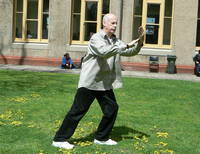Like   Tweet   Pin   +1   in/* styles */ Tips & resources on wellbeing & performance   ||   Issue 42, April 2022
 table div table+table+table div table{width:100%;padding:0}table div table+table+table div table img{width:96.23%;padding:0;float:none}table div table+table+table div table td{width:100%;padding:0 1.88% 18px}/* styles */Welcome to Good Chi News. In this issue I cover training methods I use, a Tai Chi Tip, a teaching method to help students perform better and a Lao Tzu quote.

Regards
Chris Bennett
Chris Chi

 table div table+table+table+table+table div table{width:100%;padding:0}table div table+table+table+table+table div table img{width:96.23%;padding:0;float:none}table div table+table+table+table+table div table td{width:100%;padding:0 1.88% 18px}/* styles */## Training RecoveryIt's important for me that I build in recovery time when training because, of the amount of time I practice my Tai Chi throughout a day. Otherwise I tend to feel mentally and physically tired, which defeats the purpose of training - to feel calm and energised.

I train six days a week and have a day off on Sunday. I also spread my training over the whole day, which allows time to recover before each set of skills. This is necessary because some of the skills are very demanding, such as high, medium and low stances.

If one does not leave time for recovery from physical, psychological or emotional effort, then stress and burn out is more than likely.

Image courtesy of Martin Sanchez via Unsplash

 table div table+table+table+table+table+table+table+table div table{width:100%;padding:0}table div table+table+table+table+table+table+table+table div table img{width:96.23%;padding:0;float:none}table div table+table+table+table+table+table+table+table div table td{width:100%;padding:0 1.88% 18px}/* styles */## Training EnvironmentThe ideal training environment is outdoors, with level ground and a shaded area with moderate temperature. If possible I'll train in my back yard (a treed open area) or find a park

However these conditions or circumstances aren't always available, in which case I do my training inside my living room and/or garage.

Courtesy of Beatrice Selly via Unsplash

 table div table+table+table+table+table+table+table+table+table+table+table div table{width:100%;padding:0}table div table+table+table+table+table+table+table+table+table+table+table div table img{width:96.23%;padding:0;float:none}table div table+table+table+table+table+table+table+table+table+table+table div table td{width:100%;padding:0 1.88% 18px}/* styles */## Tai Chi Tip

 /* styles */ Top half of your body should be loose and the bottom half grounded.
 table div table+table+table+table+table+table+table+table+table+table+table+table+table+table div table{width:100%;padding:0}table div table+table+table+table+table+table+table+table+table+table+table+table+table+table div table img{width:96.23%;padding:0;float:none}table div table+table+table+table+table+table+table+table+table+table+table+table+table+table div table td{width:100%;padding:0 1.88% 18px}/* styles */## Let Go - Maintain StructureThe phrase 'let go but maintain your structure' is one I often repeat when teaching Tai Chi.

I do this because it helps to prevent students from relaxing so much that they lose the correct structure required.

Incorrect structure leads to poor performance, lack of chi flow and possible injury.

 table div table+table+table+table+table+table+table+table+table+table+table+table+table+table+table+table+table div table{width:100%;padding:0}table div table+table+table+table+table+table+table+table+table+table+table+table+table+table+table+table+table div table img{width:96.23%;padding:0;float:none}table div table+table+table+table+table+table+table+table+table+table+table+table+table+table+table+table+table div table td{width:100%;padding:0 1.88% 18px}/* styles */table div table+table+table+table+table+table+table+table+table+table+table+table+table+table+table+table+table+table+table div table{width:100%;padding:0}table div table+table+table+table+table+table+table+table+table+table+table+table+table+table+table+table+table+table+table div table img{width:96.23%;padding:0;float:none}table div table+table+table+table+table+table+table+table+table+table+table+table+table+table+table+table+table+table+table div table td{width:100%;padding:0 1.88% 18px}/* styles *//* styles */
 table div table+table+table+table+table+table+table+table+table+table+table+table+table+table+table+table+table+table+table+table+table div table{width:100%;padding:0}table div table+table+table+table+table+table+table+table+table+table+table+table+table+table+table+table+table+table+table+table+table div table img{width:96.23%;padding:0;float:none}table div table+table+table+table+table+table+table+table+table+table+table+table+table+table+table+table+table+table+table+table+table div table td{width:100%;padding:0 1.88% 18px}/* styles *//* styles */